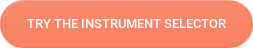Density is a physical property of matter that is defined as the mass of a material per its unit volume.

## What is density?

Density is the mass of a material per its unit volume as follows:

ρ = m/V

Where ρ is the density of the object (kg/m3), m is the mass of the object (kg) and V is the volume of the object (m3). Density defines the tightness of the particles packed inside the substance. The tighter the particles are packed, the higher is the density of the material. Density is a bulk property, which does not depend on the size or amount of the material. Density of a material remains constant at constant temperature. Different substances have different densities, which enables the identification of an unknown material through the evaluation of its density.

## How is density measured?

The Attension® Sigma force tensiometer together with the density kit can be used to measure the density of an unknown liquid. The density of a liquid is important to know in many different physical applications.

## Video of a density measurement using the Sigma 700 force tensiometer# Let's find the best tensiometer for your measurements!

Our tensiometer selector guides you to your optimal instrument.